Скачать презентацию Price competition Firm Behavior under Profit Maximization

3c3480771eeaaf32f1c668f6ee574c2f.ppt

• Количество слайдов: 21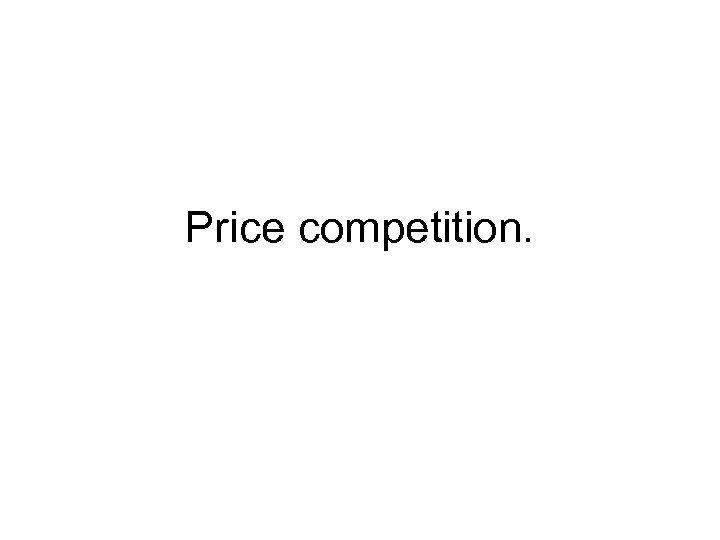Price competition.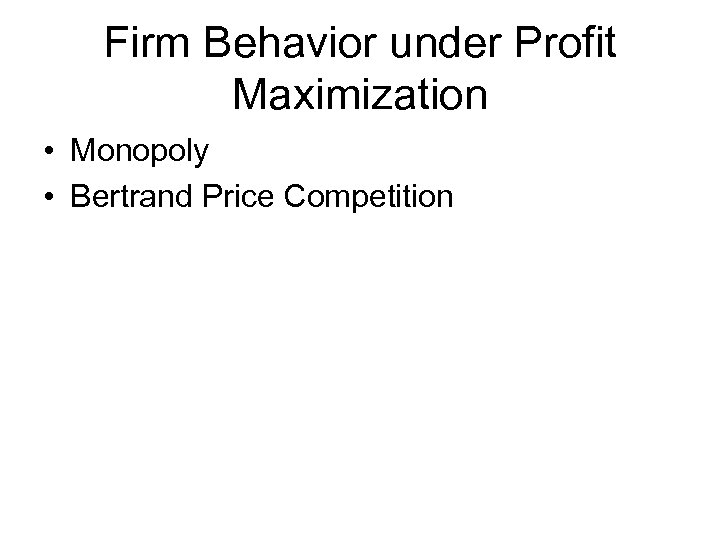Firm Behavior under Profit Maximization • Monopoly • Bertrand Price Competition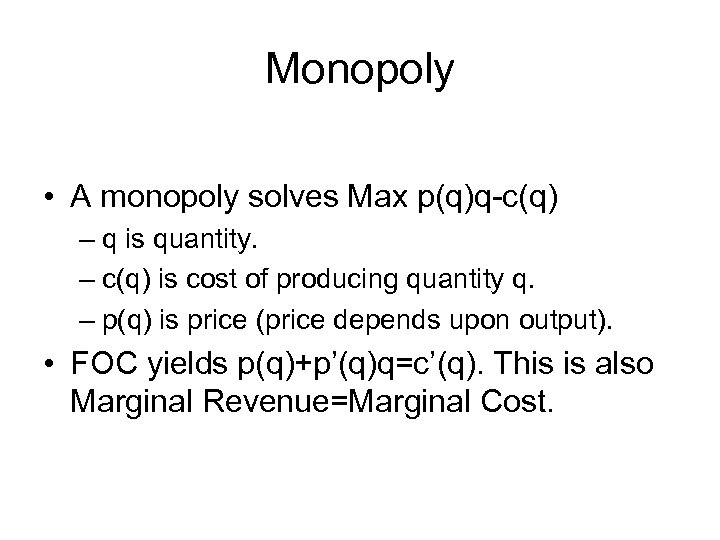Monopoly • A monopoly solves Max p(q)q-c(q) – q is quantity. – c(q) is cost of producing quantity q. – p(q) is price (price depends upon output). • FOC yields p(q)+p’(q)q=c’(q). This is also Marginal Revenue=Marginal Cost.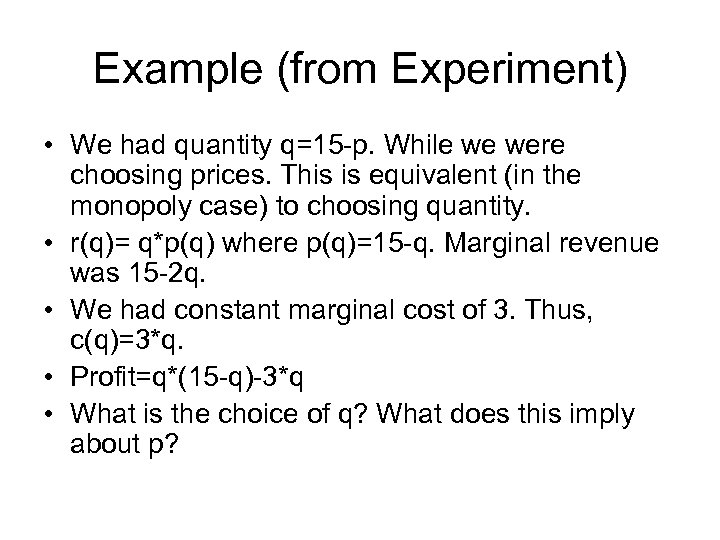Example (from Experiment) • We had quantity q=15 -p. While we were choosing prices. This is equivalent (in the monopoly case) to choosing quantity. • r(q)= q*p(q) where p(q)=15 -q. Marginal revenue was 15 -2 q. • We had constant marginal cost of 3. Thus, c(q)=3*q. • Profit=q*(15 -q)-3*q • What is the choice of q? What does this imply about p?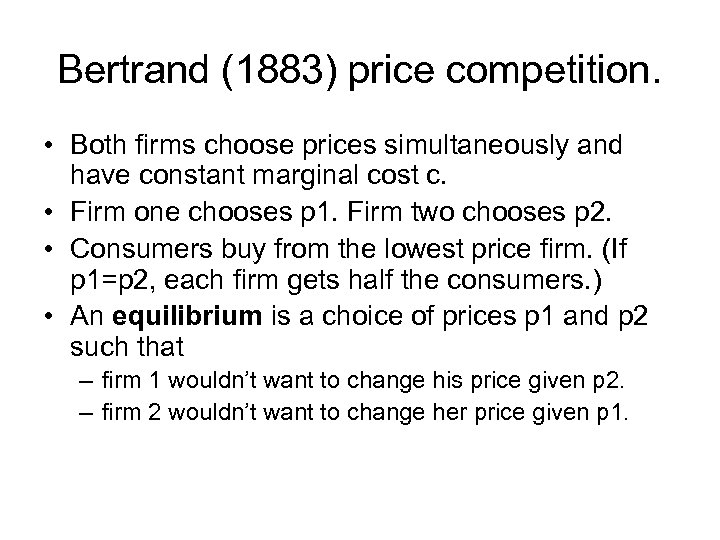Bertrand (1883) price competition. • Both firms choose prices simultaneously and have constant marginal cost c. • Firm one chooses p 1. Firm two chooses p 2. • Consumers buy from the lowest price firm. (If p 1=p 2, each firm gets half the consumers. ) • An equilibrium is a choice of prices p 1 and p 2 such that – firm 1 wouldn’t want to change his price given p 2. – firm 2 wouldn’t want to change her price given p 1.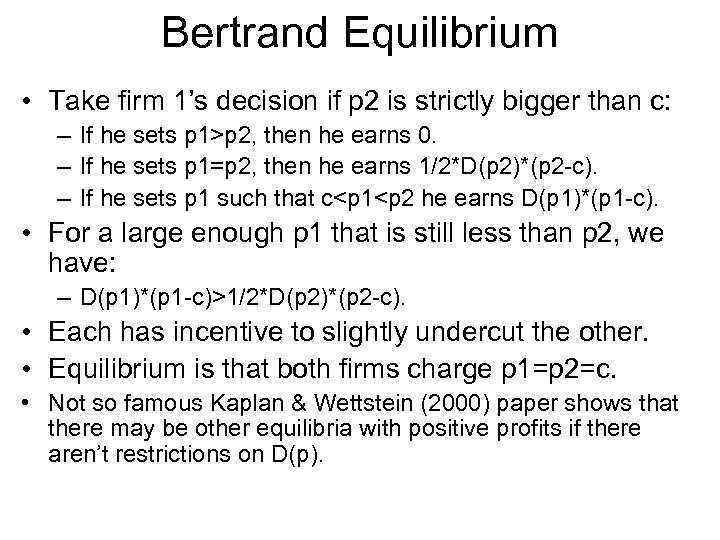Bertrand Equilibrium • Take firm 1’s decision if p 2 is strictly bigger than c: – If he sets p 1>p 2, then he earns 0. – If he sets p 1=p 2, then he earns 1/2*D(p 2)*(p 2 -c). – If he sets p 1 such that c

1/2*D(p 2)*(p 2 -c). • Each has incentive to slightly undercut the other. • Equilibrium is that both firms charge p 1=p 2=c. • Not so famous Kaplan & Wettstein (2000) paper shows that there may be other equilibria with positive profits if there aren’t restrictions on D(p).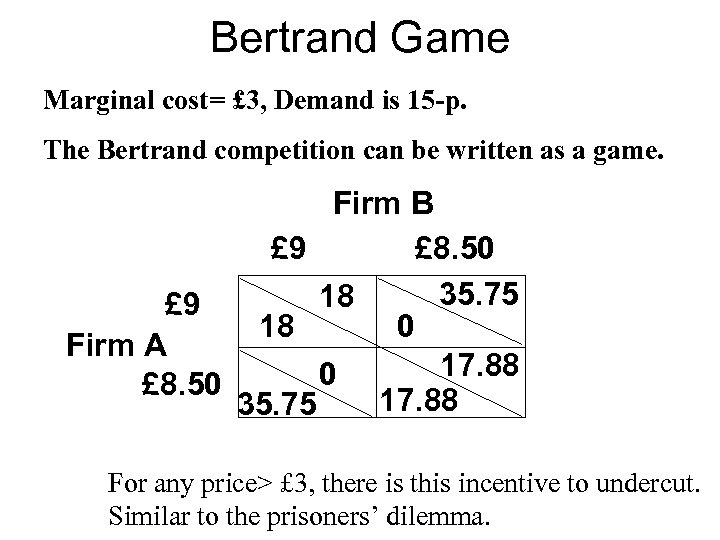Bertrand Game Marginal cost= £ 3, Demand is 15 -p. The Bertrand competition can be written as a game. Firm B £ 9 £ 8. 50 35. 75 18 £ 9 18 0 Firm A 17. 88 0 £ 8. 50 17. 88 35. 75 For any price> £ 3, there is this incentive to undercut. Similar to the prisoners’ dilemma.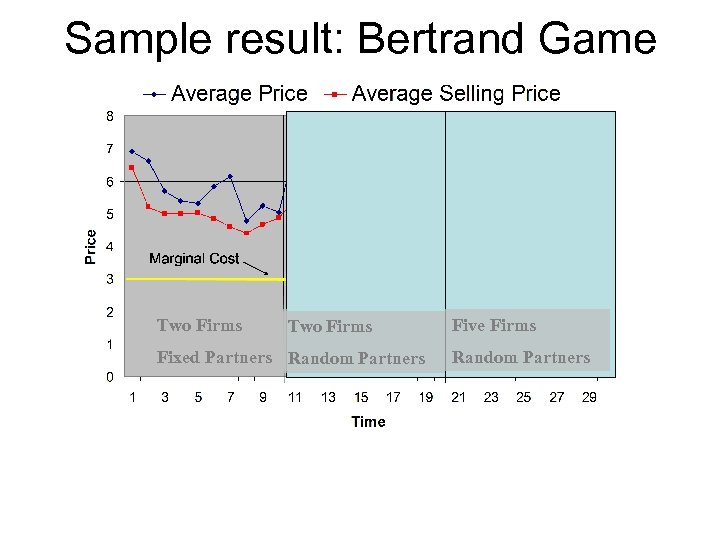Sample result: Bertrand Game Two Firms Fixed Partners Random Partners Five Firms Random Partners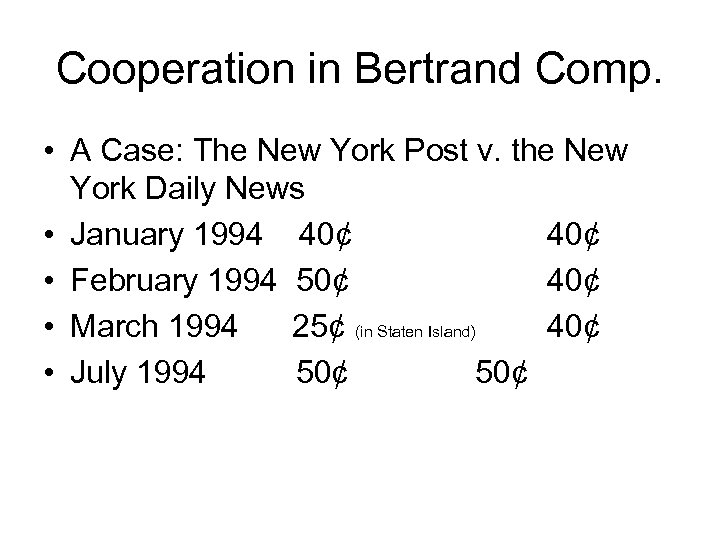Cooperation in Bertrand Comp. • A Case: The New York Post v. the New York Daily News • January 1994 40¢ • February 1994 50¢ 40¢ • March 1994 25¢ (in Staten Island) 40¢ • July 1994 50¢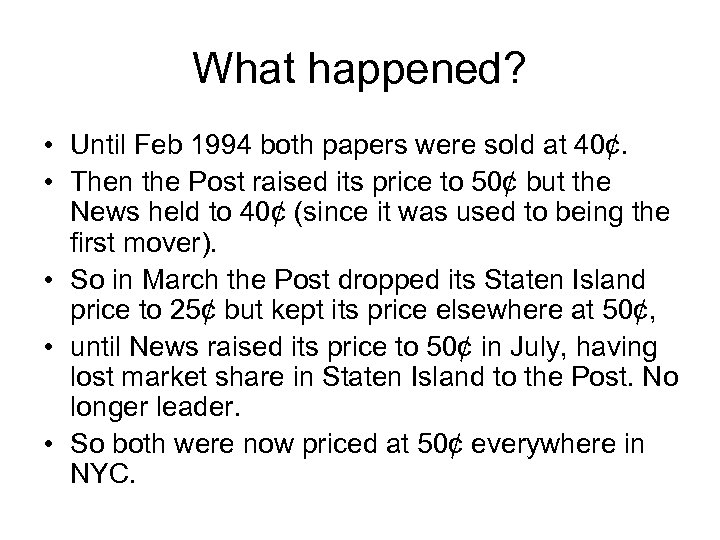What happened? • Until Feb 1994 both papers were sold at 40¢. • Then the Post raised its price to 50¢ but the News held to 40¢ (since it was used to being the first mover). • So in March the Post dropped its Staten Island price to 25¢ but kept its price elsewhere at 50¢, • until News raised its price to 50¢ in July, having lost market share in Staten Island to the Post. No longer leader. • So both were now priced at 50¢ everywhere in NYC.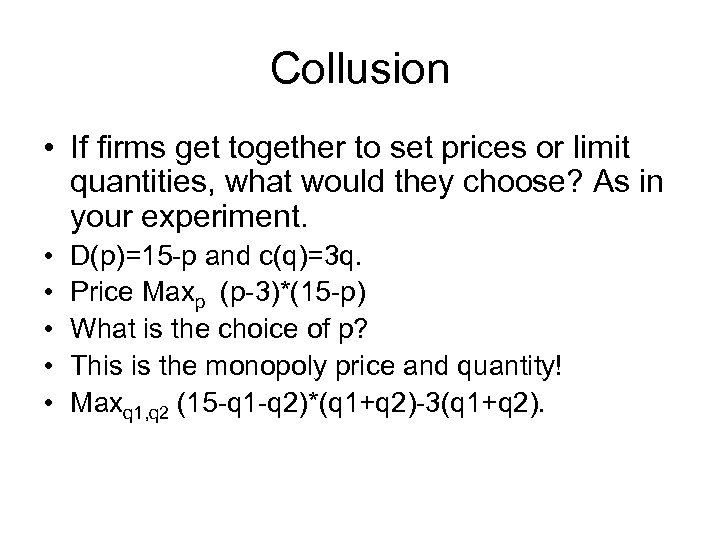Collusion • If firms get together to set prices or limit quantities, what would they choose? As in your experiment. • • • D(p)=15 -p and c(q)=3 q. Price Maxp (p-3)*(15 -p) What is the choice of p? This is the monopoly price and quantity! Maxq 1, q 2 (15 -q 1 -q 2)*(q 1+q 2)-3(q 1+q 2).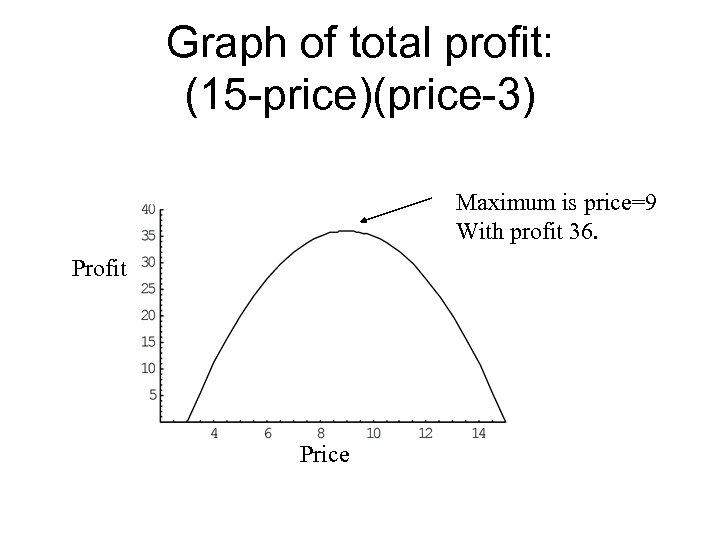Graph of total profit: (15 -price)(price-3) Maximum is price=9 With profit 36. Profit Price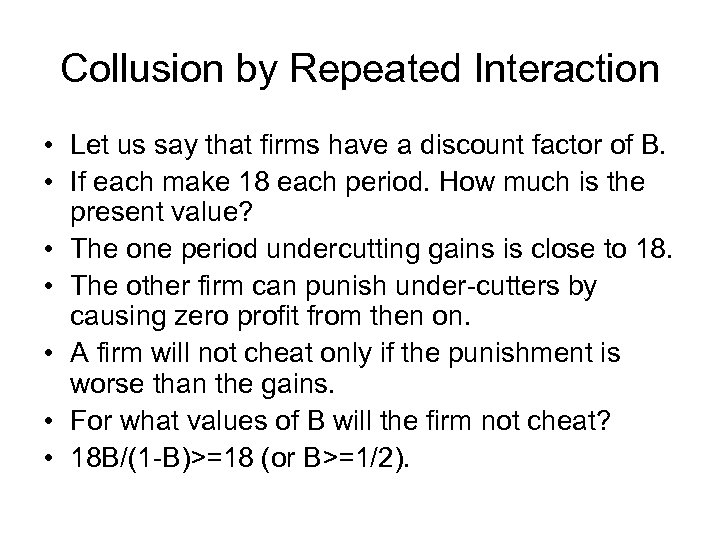Collusion by Repeated Interaction • Let us say that firms have a discount factor of B. • If each make 18 each period. How much is the present value? • The one period undercutting gains is close to 18. • The other firm can punish under-cutters by causing zero profit from then on. • A firm will not cheat only if the punishment is worse than the gains. • For what values of B will the firm not cheat? • 18 B/(1 -B)>=18 (or B>=1/2).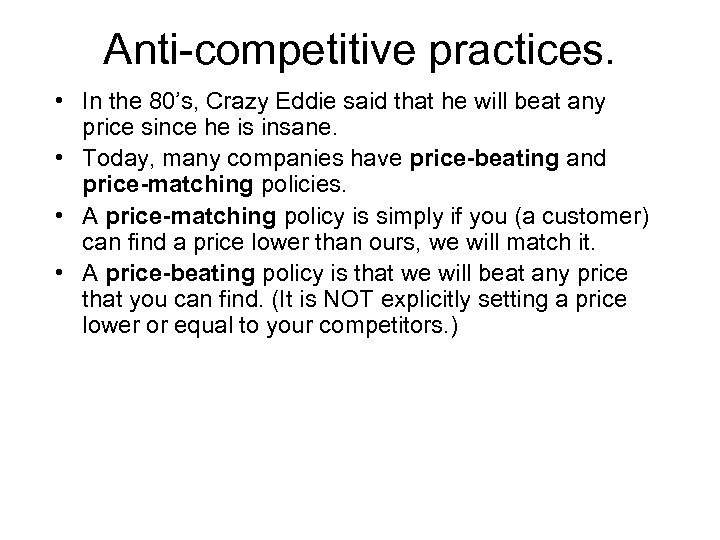Anti-competitive practices. • In the 80’s, Crazy Eddie said that he will beat any price since he is insane. • Today, many companies have price-beating and price-matching policies. • A price-matching policy is simply if you (a customer) can find a price lower than ours, we will match it. • A price-beating policy is that we will beat any price that you can find. (It is NOT explicitly setting a price lower or equal to your competitors. )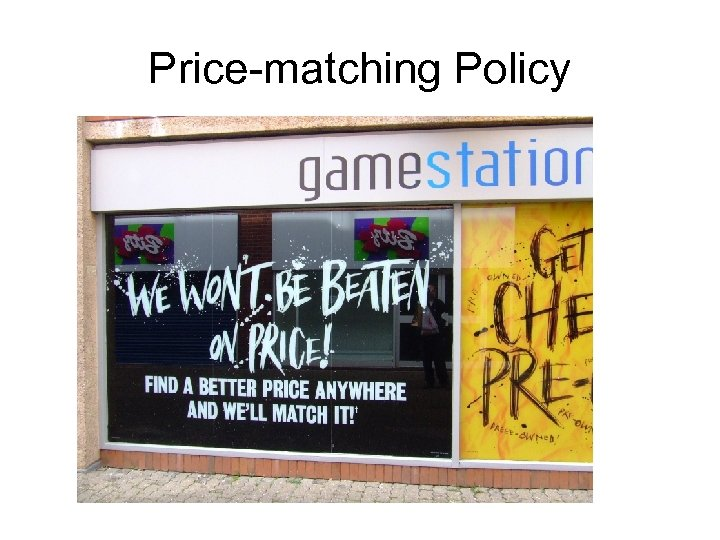Price-matching Policy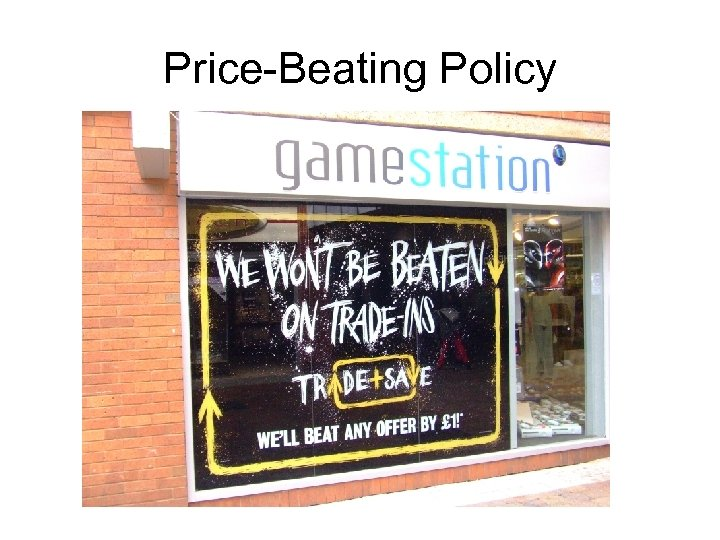Price-Beating Policy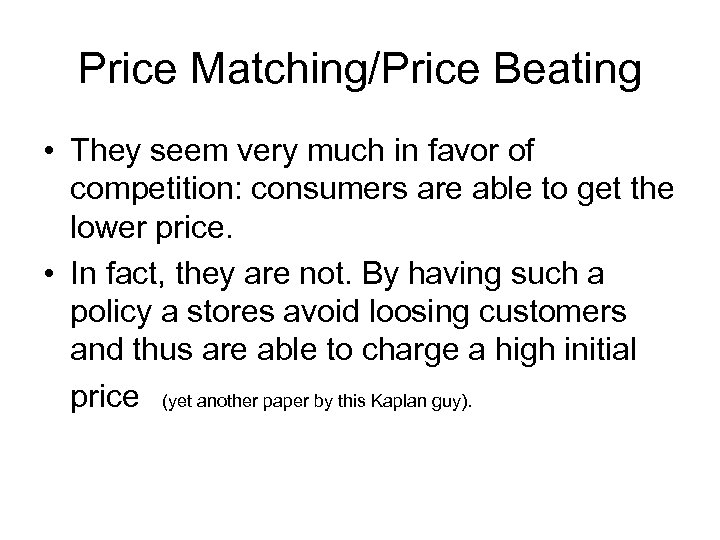Price Matching/Price Beating • They seem very much in favor of competition: consumers are able to get the lower price. • In fact, they are not. By having such a policy a stores avoid loosing customers and thus are able to charge a high initial price (yet another paper by this Kaplan guy).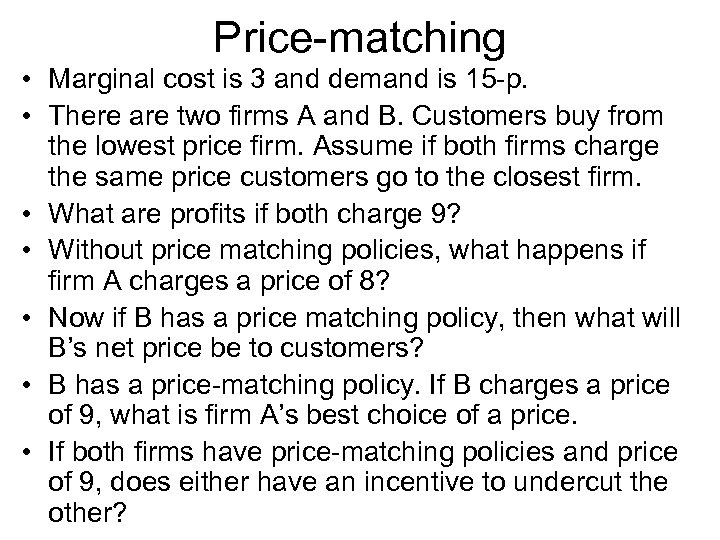Price-matching • Marginal cost is 3 and demand is 15 -p. • There are two firms A and B. Customers buy from the lowest price firm. Assume if both firms charge the same price customers go to the closest firm. • What are profits if both charge 9? • Without price matching policies, what happens if firm A charges a price of 8? • Now if B has a price matching policy, then what will B’s net price be to customers? • B has a price-matching policy. If B charges a price of 9, what is firm A’s best choice of a price. • If both firms have price-matching policies and price of 9, does either have an incentive to undercut the other?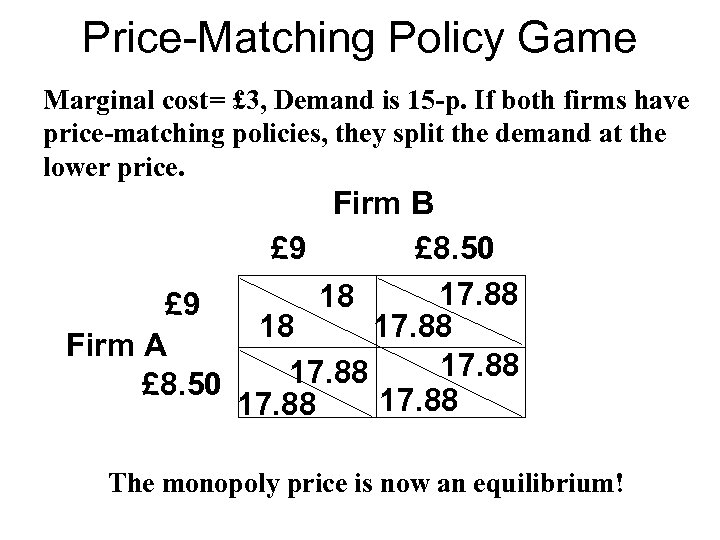Price-Matching Policy Game Marginal cost= £ 3, Demand is 15 -p. If both firms have price-matching policies, they split the demand at the lower price. Firm B £ 9 £ 8. 50 17. 88 18 £ 9 18 17. 88 Firm A 17. 88 £ 8. 50 17. 88 The monopoly price is now an equilibrium!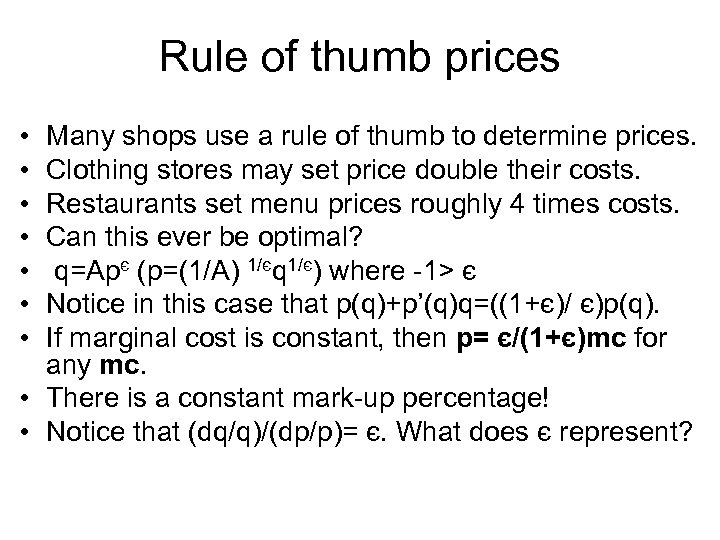Rule of thumb prices • • Many shops use a rule of thumb to determine prices. Clothing stores may set price double their costs. Restaurants set menu prices roughly 4 times costs. Can this ever be optimal? q=Apє (p=(1/A) 1/єq 1/є) where -1> є Notice in this case that p(q)+p’(q)q=((1+є)/ є)p(q). If marginal cost is constant, then p= є/(1+є)mc for any mc. • There is a constant mark-up percentage! • Notice that (dq/q)/(dp/p)= є. What does є represent?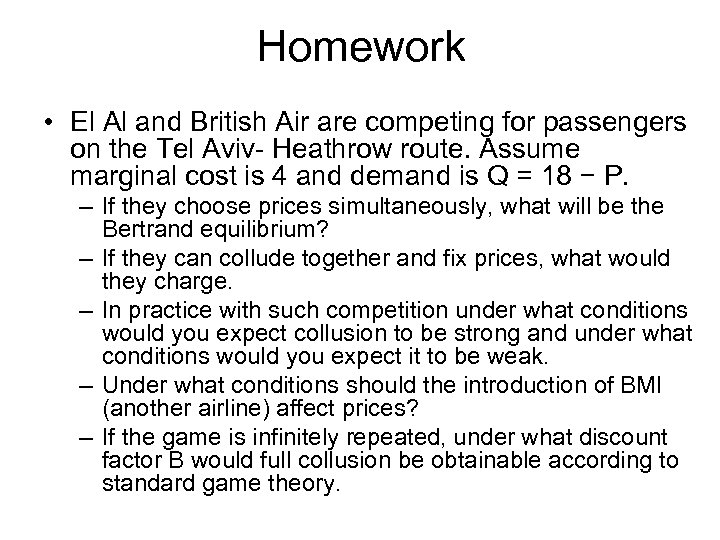Homework • El Al and British Air are competing for passengers on the Tel Aviv- Heathrow route. Assume marginal cost is 4 and demand is Q = 18 − P. – If they choose prices simultaneously, what will be the Bertrand equilibrium? – If they can collude together and fix prices, what would they charge. – In practice with such competition under what conditions would you expect collusion to be strong and under what conditions would you expect it to be weak. – Under what conditions should the introduction of BMI (another airline) affect prices? – If the game is infinitely repeated, under what discount factor B would full collusion be obtainable according to standard game theory.